#### You may also like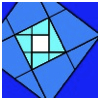### Extending Great Squares

Explore one of these five pictures.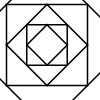### Shaping It

These pictures were made by starting with a square, finding the half-way point on each side and joining those points up. You could investigate your own starting shape.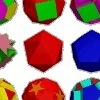### Cylinder Cutting

An activity for high-attaining learners which involves making a new cylinder from a cardboard tube.

# Area and Perimeter

##### Age 7 to 11Challenge Level

Matthew from Parkgate Primary School focused on the first two shapes in the problem. He said:

The area of the rectangle is $18$cm$^2$ and its perimeter is $18$cm.
The second shape is $12$cm$^2$ and its perimeter is $22$cm.

Thomas from Colet Court examined the eight shapes which were drawn on the cards.  He labelled the shapes A, B, C, D, E, F, G and H, going from left to right in the top row, then left to right in the bottom row.

A: P is 18 , A is 18; B: P is 20 , A is 17; C: P is 18 , A is 16; D: P is 20 , A is 16
E: P is 22 , A is 12; F: P is 12 , A is 9; G: P is 20 , A is 25; H: P is 16 , a is 9

Thomas didn't give any units in his solution.  I guess we could say the perimeter is measured in 'units' and the area in 'square units', although some of you, like Matthew above, assumed the squares were 1cm long.  So, that would mean the perimeter is in cm and the area in cm$^2$. Thomas remarked:

The perimeter is always bigger except for one (Shape G).

Noor from Kingsbury Green Primary School answered the question; 'Can you draw a shape in which the area is numerically equal to its perimeter? And another?'.  He said:

I found if I did $4\times4$ I would get an area of $16$.
If I counted the sides there would be four on each side: $4+4+4+4 = 16$.
The area and perimeter are the same.

The same happened if there you have a rectangle that has a length of $6$ and a width of $3$.

Table 3 (they didn't give their school) looked at finding a shape which has a perimeter numerically twice the area.  They wrote:

Yes you can draw a the shape in which the perimeter is numerically twice the area, it is a $2$ by $2$ square, because the area is $4$cm$^2$ and the perimeter is $8$cm.

Bashayer from Kingsbury Green Primary also found this solution.  Miiti from Kingsbury Green created this shape which has a perimeter of $20$ units and an area of $10$ square units: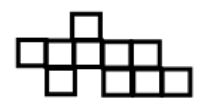Thomas from Colet Court drew a shape in which the area is numerically twice the perimeter: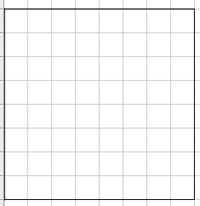Thomas went on to investigate how to make the area of a shape go up but the perimeter go down.  He said:

...you go from a dented square to a square shape.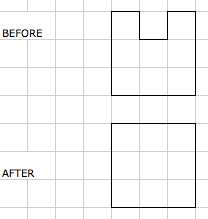Thomas  also said that you can make the perimeter of a shape go up but the area go down

by inserting a dent in your shape the area gets reduced by the dent.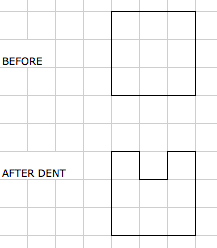Joe and Charlie from Coniston Primary described the way they worked on this part of the problem:

First we picked a shape which was a square so we looked at the area and perimeter.
The area was $25$cm$^2$ and the perimeter was $20$cm.
We took $1$ chunk out of the top of the square and it did make the perimeter bigger and the area became smaller. The perimeter became bigger because it adds on $2$ more lines so the perimeter became $22$cm.

Whilst investigating problem 7280, Anna from Air Balloon Hill Primary made the following observations which she would like to share with you. Please see attachment taken from her exercise book.  Year 5 teacher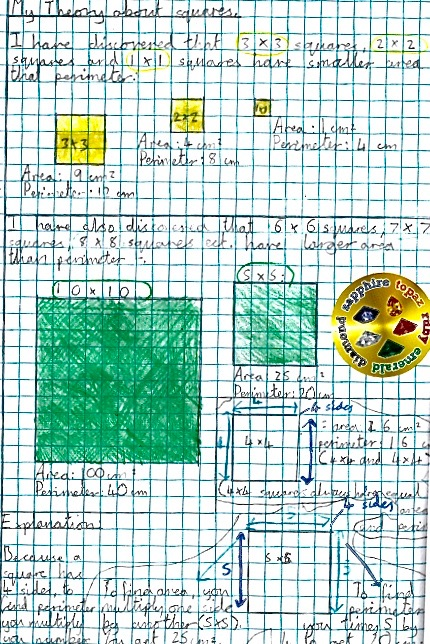Very, very well done all of you.  You have obviously put a lot of thought into this problem.